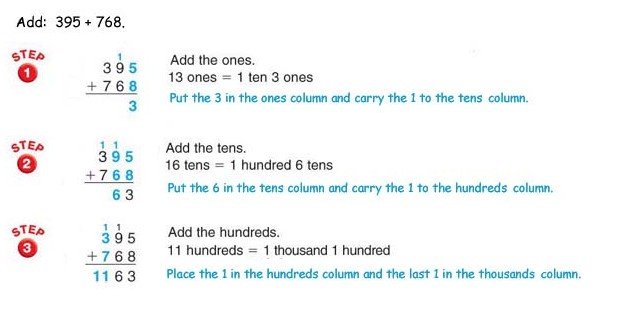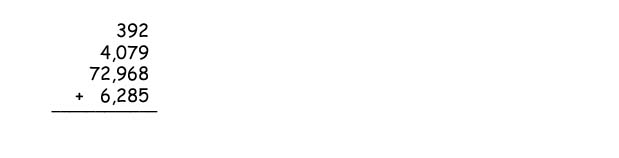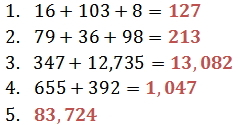Addition is the process of finding the total, or sum, of a set of numbers (2 or more).

\$113   +   \$40   =    \$153

Some special properties of addition are given below.

Working with Large Numbers

To add larger numbers, begin by arranging the numbers vertically, keeping the digits of the same place value in the same column.

When the sum of the digits in a column exceeds 9, the addition will involve "carrying."There are several key words or phrases in English that show the need to use addition. Here are some examples:

 Phrase Example Number Sentence added to 3 added to 5 5 + 3 more than 4 more than 6 6 + 4 increased by 5 increased by 2 5 + 2 the sum of the sum of 3 and 9 3 + 9 the total of the total of 7 and 10 7 + 10

Practice

1. Find the sum of 16; 103; and 8.

2. Add: 79 + 36 + 98

3. What is 347 increased by 12,735?

4. Find the sum of 392 and 655.